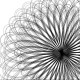Don't Miss

# Neural network and astronomy

By on 28/02/2017The following article was previously published on DocMadhattan. There is also an Italian version published on MaddMaths!

Neural network is one of the most powered method to analize data. It can be use in most research subject, for example in astronomy: in this case, we can use NNs to examine astronomical images or also the red shift effect. For example, in 2003 Jorge Núñez (Universitad de Barcelona) and Jorge Llacer (EC Engineering Consultants LLC) published a paper Núñez J. & Llacer J. (2003). Astronomical image segmentation by self-organizing neural networks and wavelets, Neural Networks, 16 (3-4) 411-417. DOI: 10.1016/S0893-6080(03)00011-X in which they describe the develop of an algorithm to study astronomical image segmentation that uses a self-organizing neural network as basis. In their work, the scientists examine the separation between some stars and also a Saturn’s image: the alghoritm seems quite robust against noise and fragmentation.
In the same year, a group of italian astronomers published a review Tagliaferri R., Longo G., Milano L., Acernese F., Barone F., Ciaramella A., Rosa R.D., Donalek C., Eleuteri A., Raiconi G. & Sessa S. (2003). Neural neZtworks in astronomy, Neural Networks, 16 (3-4) 297-319. DOI: (pdf) of the models used in astronomy and examined some data used by AstroNeural collaboration. Finally in 2004 a collaboration between researchers in Italy, Germany and France perform an application of NNs to redshift calculations Vanzella E., Cristiani S., Fontana A., Nonino M., Arnouts S., Giallongo E., Grazian A., Fasano G., Popesso P. & Saracco P. & (2004). Photometric redshifts with the Multilayer Perceptron Neural Network: Application to the HDF-S and SDSS, Astronomy and Astrophysics, 423 (2) 761-776. DOI: (arXiv).Now you can quest: What is a neural network?
It is a system of node and link. In the structure we can eventually distinguished between different layers, and every layers are fully connected. Such networks are usually called <e,>Multilayer Perceptron NN, and the interaction between every node is modelled by the following funcition
$f(x) = k \sum_i w_i g_i(x)$ where $k$ is some predefined function, $g_i$ is a vector of functions.
A more simple model is
$n_j = h \sum_i w_{ij} z_i$ where $n_j$ is the $j$-th node, $h$ is a constant, $w_{ij}$ the weights of the link, $z_i$ the previous node.
There is also the self-organizing maps, in which every node, or neurons, are in competition with each other:It’s possible implement NNs with fuzzy logic and perform image segmentation, object detection, noise identification (for example in detection of gravitational waves), estimation of redshifts. The great advantage using NNs in astronomy is, however, to perform complex calculations with usually alghoritms. For example, we can see the plots in Vanzella’s et al. paper(3). In the work, astronomers used a Multilayer Perceptron NN, using real data from Hubble Deep Field North dataset.This is the first comparison between

spectroscopic redshift in the HDF-S and the neural redshift using the colors as an input pattern. The training has been done on the HDF-N spectroscopic sample, the estimation of the redshift for each object is the median of 100 predictions and the error bars represent 1- interval. Open circles represent objects with unreliable photometry and triangles are objects with uncertain spectroscopic redshift.And here there is a comparison after adding informations.
One of the most important tool in NNs is the ability of these type of networks to learn from data.
The last plot that I propose you is the redshift distribution of the spectroscopic sample:And now some tutorials: How to build a brain with neural networks and, for all geek, Evolving Neural Networks with SharpNEAT: part 1 and part 2. And, in conclusion, read the following post about NNs and tea leaves.
Enjoy!

Gianluigi Filippelli

References   [ + ]

 1 ↑ Núñez J. & Llacer J. (2003). Astronomical image segmentation by self-organizing neural networks and wavelets, Neural Networks, 16 (3-4) 411-417. DOI: 10.1016/S0893-6080(03)00011-X 2 ↑ Tagliaferri R., Longo G., Milano L., Acernese F., Barone F., Ciaramella A., Rosa R.D., Donalek C., Eleuteri A., Raiconi G. & Sessa S. (2003). Neural neZtworks in astronomy, Neural Networks, 16 (3-4) 297-319. DOI: 10.1016/S0893-6080(03)00028-5 (pdf) 3 ↑ Vanzella E., Cristiani S., Fontana A., Nonino M., Arnouts S., Giallongo E., Grazian A., Fasano G., Popesso P. & Saracco P. & (2004). Photometric redshifts with the Multilayer Perceptron Neural Network: Application to the HDF-S and SDSS, Astronomy and Astrophysics, 423 (2) 761-776. DOI: 10.1051/0004-6361:20040176 (arXiv)